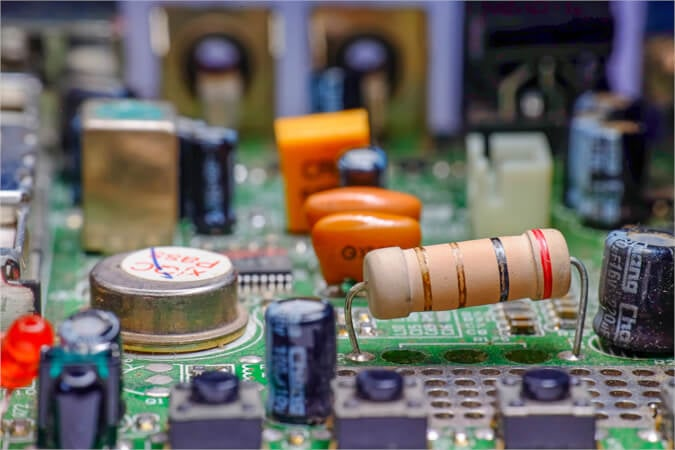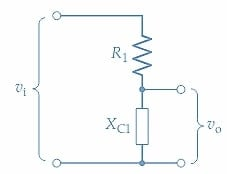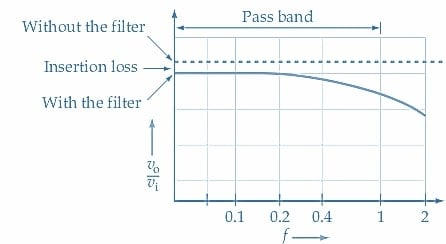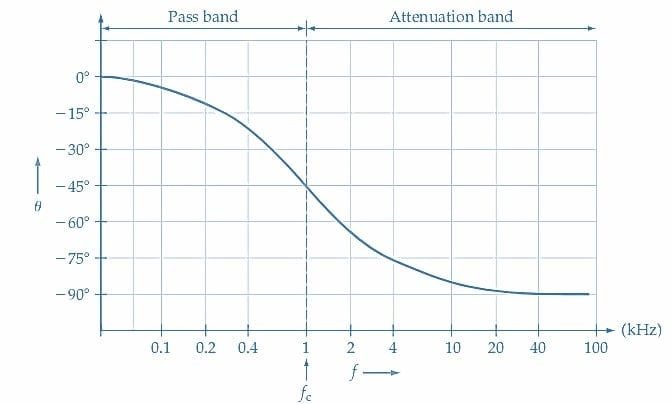Technical Article

# Calculating RC Low-Pass Filter Cut-Off Frequency and Transfer Function

July 18, 2023 by Amna Ahmad

## Learn how to determine the RC low-pass filter's cut-off frequency and transfer function and plot the gain/frequency and phase/frequency response graphs.

An RC low-pass filter circuit allows low-frequency signals to pass through while attenuating high-frequency signals. It consists of a resistor (R) and a capacitor (C) connected in series. The filter operation is based on the time constant of the RC circuit, which determines the rate at which the capacitor charges and discharges.##### Image used courtesy of Adobe Stock

Figure 1(a) shows a basic RC low-pass filter circuit (R1 and C1) together with a signal voltage (vi) and a load resistor (RL). Resistance R1 and capacitive reactance XC1 constitute a voltage divider, as illustrated in Figure 1(b). When the filter input voltage (vi) has a low frequency (f), the capacitor impedance $$\Bigg[X_{C1}=\frac{1}{2\pi fC_{1}}\Bigg]$$ is much larger than the resistance of R1. In this case, there is very little voltage division, and the output voltage (vo) approximately equals the input. When vi has a high frequency, XC1 becomes much smaller than R1, and so vo becomes much smaller than vi. It is seen that low-frequency input voltages are passed to the output, and high-frequency inputs are attenuated.##### (a) RC low-pass filter circuit### Gain/Frequency Response

The typical graph of output-to-input voltage ratio $$(\frac{v_{_o}}{v_{_i}}\,in\,decibels)$$ plotted versus input frequency (f) for a low-pass filter is shown in Figure 2(a). This is the filter gain/frequency response graph (Bode plot). The output remains substantially equal to the input $$(v_{_o}/v_{_i}\approx1\approx0dB)$$ for all frequencies up to the cut-off frequency (fc). This is the upper limit of the passband for the filter, and all frequencies above fc are in the attenuation band, as illustrated.

The cut-off frequency is when the output voltage falls 3 dB from its normal (low frequency) level. It is also referred to as the 3 dB and critical frequencies. The cut-off frequency can be expected to occur when XC1 becomes equal to the resistance of R1.

$X_{C1}=\frac{1}{2\pi_{c}C_{1}}=R_{1}$

Resulting in

$f_{c}=\frac{1}{2\pi R_{_1}C_{_1}}(1)$

Equation 1 is correct only when the resistance of R1 is much smaller than the load resistance (R1<L in Figure 1). When R1 is not smaller than RL, then fc occurs when XC1 equals R1 ǁ RL

An equation for the ratio of output-to-input voltage for the RC low-pass filter is easily derived from the voltage divider in Figure 1(b):

$\frac{v_{_o}}{v_{_i}}=\frac{X_{_{C1}}}{\sqrt{R^{2}_{1}+X^{2}_{C1}}}(2)$

When XC1 = R1

$\frac{v_{_o}}{v_{_i}}=\frac{R_{1}}{\sqrt{R^{2}_{1}+R^{2}_{1}}}=\frac{1}{\sqrt{2}}=0.707$

So, XC1= R1 at fc, and this occurs when vo/vi=0.707 $$\Big(or\frac{1}{\sqrt2}\Big)$$, which is also -3 dB below its normal (low frequency) level. The output power is halved at the -3 dB point, giving the half-power point one more name for the cut-off frequency. Ideally, the low-pass filter's gain/frequency response graph should be perfectly flat below the cut-off frequency and perfectly vertical above fc to produce zero signal attenuation with frequencies within the pass band and infinite attenuation of those with frequencies in the attenuation band. This is impossible with practical circuits; however, the steepness of the frequency response graph within the attenuation band can be an important consideration for a filter.

Figure 2(a) shows that for signal frequencies above fc, the output voltage decreases at a rate of 6 dB per octave; a 6 dB reduction each time the frequency is doubled. As illustrated, this fall-off rate (also referred to as the roll-off rate) can also be stated as 20 dB per decade, a 20 dB reduction for ten times increase in signal frequency.##### (a) Gain/frequency response for low-pass filter### Insertion Loss

For signal frequencies within the filter passband, there is some attenuation of the input, known as the insertion loss, as illustrated in Figure 2(b). The insertion loss is defined as the loss of signal due to the presence of the filter, and for the filter circuit in Figure 1, it is simply the result of voltage division across R1 and RL. The insertion loss can be determined by measuring the output (load) voltage without the filter in the circuit and measuring it again with the filter connected. The measured quantities are then used to calculate the loss in decibels. The insertion loss can also be determined from the filter component and load resistance values.

### Phase/Frequency Response

Because the low-pass filter output is the capacitor voltage, there is a phase shift between the input and output voltages. The phase/frequency response of the filter is a plot of the phase difference (θ) between the input and output voltages versus signal frequency (f), (see Figure 3). The phase shift is seen to be zero at low signal frequencies where XC1>>R1. As the signal frequency increases, the (lagging) phase shift gradually increases to -45° at fc, and then continues beyond fc to a maximum of -90°. The phase angle (θ) between the input and capacitor (filter output) voltages is (90°- φ), and it can also be determined directly as,

$\theta=\Big[\frac{-R1}{X_{C1}}\Big](3)$

At fc, where XC1=R1, θ is calculated from Equation 3 as -45°.### Low-Pass Filter Transfer Function

Combining Equations 2 and 3 gives the transfer function for the filter, which is the phasor input-output relationship.

$\frac{v_{_o}}{v_{_i}}=\frac{X_{C1}}{\sqrt{R^{2}_{1}+X^{2}_{C1}}}\angle tan \,\^{}-1\Big(\frac{-R_{1}}{X_{C1}}\Big)(4)$

Substituting

$R_{1}=\frac{1}{2\pi f_{C}C_{1}},andX_{C1}=\frac{1}{(2\pi f_{C1})}$

Equation 4 can be simplified to

$\frac{v_{_o}}{v_{_i}}=\Bigg(\frac{1}{\sqrt{(\frac{f}{f_{c}})^{2}+1}}\Bigg)\angle tan\,\^{}-1(\frac{-f}{f_{c}})(5)$

Expressing $$\frac{v_{_o}}{v_{_i}}$$ in decibels

$\frac{v_{_o}}{v_{_i}}=20log\Bigg(\frac{1}{\sqrt{(\frac{f}{f_{c}})^{2}+1}}\Bigg)\angle tan\,\^{}-1(\frac{-f}{f_{c}})(6)$

Once fc is calculated for a given RC low-pass filter, Equation 6 can be used to quickly determine the values of vo/vi and θ at various frequencies (multiples of fc) for plotting the gain/frequency and phase/frequency responses.

### RC Low-Pass Filter Takeaways

The RC low-pass filter circuit consists of a resistor and a capacitor, which work together to attenuate high-frequency signals while allowing low-frequency signals to pass through. The filter's cut-off frequency can be determined using the values of the resistor and capacitor. The transfer function is the relationship between the input and output signals of the filter, and the gain/frequency and phase/frequency response graphs provide a visual representation of how the filter affects different frequencies.